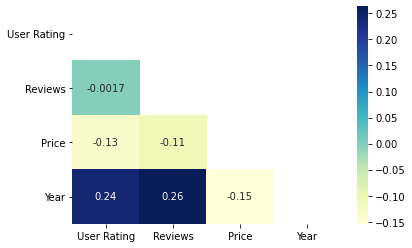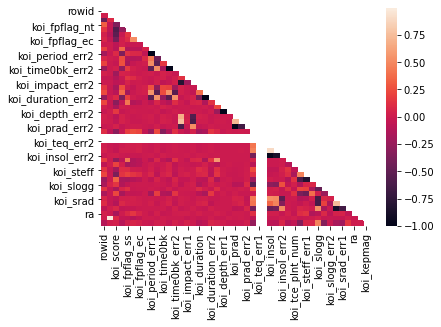Related Articles
How to create a Triangle Correlation Heatmap in seaborn – Python?
• Last Updated : 12 Nov, 2020

Seaborn is a Python library that is based on matplotlib and is used for data visualization. It provides a medium to present data in a statistical graph format as an informative and attractive medium to impart some information. A heatmap is one of the components supported by seaborn where variation in related data is portrayed using a color pallette. This article centrally focuses on a correlation heatmap and how seaborn in combination with pandas and matplotlib can be used to generate one for a dataframe.

### Installation

Like any another Python library, seaborn can be easily installed using pip:

```pip install seaborn
```

This library is a part of Anaconda distribution and usually works just by import if your IDE is supported by Anaconda, but it can be installed too by the following command:

```conda install seaborn
```

## Triangle correlation heatmap

A correlation heatmap is a heatmap that shows a 2D correlation matrix between two discrete dimensions, using colored cells to represent data from usually a monochromatic scale. The values of the first dimension appear as the rows of the table while of the second dimension as a column. The color of the cell is proportional to the number of measurements that match the dimensional value. This makes correlation heatmaps ideal for data analysis since it makes patterns easily readable and highlights the differences and variation in the same data. A correlation heatmap, like a regular heatmap is assisted by a colorbar making data easily readable and comprehensible.

A correlation heatmap is a rectangular representation of data and it repeats the same data description twice because the categories are repeated on both axis for computing analysis. Hence, the same result is obtained twice. A correlation heatmap that presents data only once without repetition that is categories are correlated only once is known as a triangle correlation heatmap. Since data is symmetric across the diagonal from left-top to right bottom the idea of obtaining a triangle correlation heatmap is to remove data above it so that it is depicted only once. The elements on the diagonal are the parts where categories of the same type correlate.

For plotting heatmap method of the seaborn module will be used. Along with that mask, argument will be passed. Mask is a heatmap attribute that takes a dataframe or a boolean array as an argument and displays only those positions which are marked as False or where masking is provided to be False.

Syntax:

heatmap(data, vmin, vmax, center, cmap,……………………………………………………)

Except for data all other attributes are optional and data obviously will be the data to be plotted. The data here has to be passed with corr() method to generate a correlation heatmap. Also, corr() itself eliminates columns which will be of no use while generating a correlation heatmap and selects those which can be used.

For masking, here an array using NumPy is being generated as shown below:

```np.triu(np.ones_like())
```

first, the ones_like() method of NumPy module will generate an array of size same as that of our data to be plotted containing only number one. Then, triu() method of the NumPy module will turn the matrix so formed into an upper triangular matrix, i.e. elements above the diagonal will be 1 and below, and on it will be 0. Masking will be applied to places where 1(True) is set.

The following steps show how a triangle correlation heatmap can be produced:

• Import all required modules first
• Import the file where your data is stored
• Plot a heatmap
• Mask the part of the heatmap that shouldn’t be displayed
• Display it using matplotlib

Example 1:

For the example given below, here a dataset downloaded from kaggle.com is being used. The plot shows data related to bestseller novels of amazon.

Dataset used – Bestsellers

## Python3

 `# import modules``import` `matplotlib.pyplot as mp``import` `pandas as pd``import` `seaborn as sb``import` `numpy as np`` ` `# import file with data``data ``=` `pd.read_csv(``"C:\\Users\\Vanshi\\Desktop\\gfg\\bestsellers.csv"``)`` ` `# creating mask``mask ``=` `np.triu(np.ones_like(data.corr()))`` ` `# plotting a triangle correlation heatmap``dataplot ``=` `sb.heatmap(data.corr(), cmap``=``"YlGnBu"``, annot``=``True``, mask``=``mask)`` ` `# displaying heatmap``mp.show()`

Output:Example 2:

The dataset used in this example is an exoplanet space research dataset compiled by nasa.

Dataset used – cumulative

## Python3

 `# import modules``import` `matplotlib.pyplot as mp``import` `pandas as pd``import` `seaborn as sb``import` `numpy as np`` ` `# import file with data``data ``=` `pd.read_csv(``"C:\\Users\\Vanshi\\Desktop\\gfg\\cumulative.csv"``)`` ` `# applying mask``mask ``=` `np.triu(np.ones_like(data.corr()))`` ` `# plotting a triangle correlation heatmap``dataplot ``=` `sb.heatmap(data.corr(), mask``=``mask)`` ` `# displaying heatmap``mp.show()`

Output:Attention geek! Strengthen your foundations with the Python Programming Foundation Course and learn the basics.

To begin with, your interview preparations Enhance your Data Structures concepts with the Python DS Course. And to begin with your Machine Learning Journey, join the Machine Learning – Basic Level Course

My Personal Notes arrow_drop_up